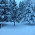## Wednesday, December 5, 2012

### Sabotage

I have this game in mind, but there's something missing.

I call the game SABOTAGE.  The game helps students to practice solving linear equations.

Download the game and instructions here.

Here is a quick rundown of the game:

Each team is given an equation that is written on the board, 4 operation cards, and 5 number cards.

On each turn the team uses one of their operation cards along with a number card to get one of the equations closer to being solved.

When an equation is finally solved the scoring goes as follows.  If x is negative, the team that "owns" that equation will get that many points.  If x is positive, then the points (value of x) is split between the team that solved the equation and the team that owns the equation.
For example, suppose that team A solves team D's equation and x = -6.  That means that team D loses 6 points.  And suppose that team B solves team C's equation and x = 3.  That means that both team B and C will receive 1.5 points.

Initially, I told the students that they could work to solve equations or sabotage equations.  What happened was a bunch of sabotaging and only one equation was solved the entire period.  So, I changed the rule so that a team must "help" and equation if possible.  If they cannot "help" an equation, then they may sabotage.  What do you think?  Or should I change it so that if they cannot "help" an equation, then they may forfeit their turn to trade in cards?

I'll try play testing again and see what happens.

Your thoughts and suggestions are MORE than welcome.

#### 1 comment:

1.Hi there!

The name of the game fits with the results :-)

If you want less sabotage you can try to disincentive it and/or increase the rewards of solving the assigned equation.

Another options could be:
- A team cannot sabotage until it has solved the assigned equation.
- They can play one round on their own, and the next on sabotaging.
- Each turn they can play both on their own and sabotaging (they will need extra cards).

Hope some of these work!
Javier